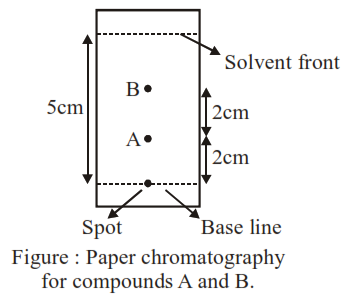# Using the provided informationQuestion:

Using the provided information in the following paper chromatogram :the calculate $R_{f}$ value of $A$_________ $\times 10^{-1}$

Solution:

$\mathrm{R}_{\mathrm{f}}=\frac{\text { Distance travelled by compound }}{\text { Distance travelled by solvent }}$

on chromatogram distance travelled by cmopound is $\rightarrow 2 \mathrm{~cm}$

Distance travelled by solvent $=5 \mathrm{~cm}$

So $\mathrm{R}_{\mathrm{f}}=\frac{2}{5}=4 \times 10^{-1}=0.4$